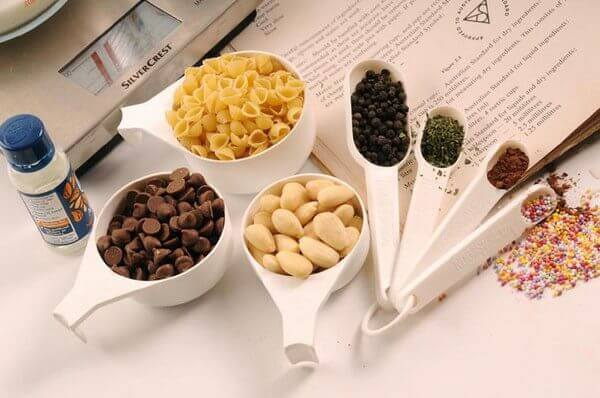# Cooking Ingredients Measurement Conversion## How do Cooking Ingredients Measurement Conversion

We found recipes in different measurements and sometimes its difficult to understand how much it is in grams, tablespoon or cups etc. This article includes Cooking Ingredients Measurement and weights of different ingredients. Now you can easily convert weight to measure and make a perfect recipe.

Liquid Measurement

• 1 teaspoon = 1/3 Tablespoon
• 1 Tbsp = 1/2 fl oz = 3 tsp
• 1 Tbsp =  1 fl oz  = 1/8 c./6 tsp
• 1 tablespoon = 15 ml = 3 teaspoons
• 1 teaspoon = 5 ml
• 1/4 c = 2 fl oz = 4 Tbsp
• 1/3 c = 2 2/3 fl oz=  5 Tbsp + 1 tsp
• 1/2 c = 4 fl oz = 8 Tbsp
• 2/3 c = 5 1/3 fl oz= 10 Tbsp + 2 tsp
• 3/4 c =  6 fl oz = 12 Tbsp
• 7/8 c = 7 fl oz = 14 Tbsp
• 1 c = 8 fl oz = 1/2 pint = 16 Tbsp
• 1 cup = 240 ml
• 1/2 cup = 120 ml
• 1/3 cup = 80 ml
• 1/4 cup= 60 ml = 4 tablespoons
• 2 c = 16 fl oz = 1 pint = 32 Tbsp
• 4 c = 32 fl oz = 2 pints = 1 quart
• 8 pints =4 quarts = 1 gallon
• 1 liter = 1.075 quarts
• 1 gallon = 128 fl oz

Spoon Measurements

• 1 level tablespoon flour = 15g flour
• 1 heaped tablespoon flour = 28g flour
• 1 level tablespoon sugar = 28g sugar
• 1 level tablespoon butter = 15g butter

Dry Ingredients Measurement

• 1 ounce = 28.35 grams
• 2 oz =  55 g
• 3 oz = 85 g
• 4 oz = 1/4 pound = 125 g
• 8 oz = 1/2 lb = 240 g
• 12 oz = 3/4 lb = 375 g
• 16 oz = 1 lb = 454 g
• 32 oz = 2 lb = 907 g
• 1 kilogram = 2.2 lb = 1000 g

Weight

• 1/4 ounce = 7 grams
• 1/2 ounce = 14 grams
• 1 ounce = 29 grams
• 1.5 ounces = 43 grams
• 2 ounces = 57 grams
• 3.5 ounces = 100 grams
• 5 ounces = 150 grams
• 7 ounces = 200 grams
• 8 ounces = 225 grams = 1/2 pound
• 10 ounces = 300 grams
• 12 ounces = 350 grams = 3/4 pound
• 14 ounces = 400 grams
• 16 ounces = 450 grams = 1 pound
• 18 ounces = 500 grams
• 24 ounces = 750 grams = 1.5 pounds
• 32 ounces = 900 grams = 2 pounds
• 64 ounces = 4 pounds = 1.8 kilograms

Weights of Common Ingredients

• All purpose flour: 1 cup = 4.5 ounces = 128 grams
• Bread flour: 1 cup = 4.8 ounces = 136 grams
• Rolled oats: 1 cup = 3 ounces = 85 grams
• White sugar: 1 cup = 7.1 ounces = 201 grams
• Packed brown sugar: 1 cup = 7.75 ounces = 22 grams

Ingredient-Specific Equivalents

• Butter
1 stick = 4 ounces = 8 tablespoons = 1/2 cup
4 sticks = 16 ounces = 32 tablespoons = 2 cups
• Chocolate
1 ounce = 1/4 cup grated
6 ounces chips = 1 cup chips
1 pound cocoa = 4 cups cocoa
• Creams
Half and half = 1/2 milk + 1/2 cream = 10.5 to 18 percent butterfat
Light cream = 18 percent butterfat
Light whipping cream = 30 to 26 percent butterfat
Heavy cream = whipping cream = 36 percent or more butterfat
Double cream = extra-thick double cream = clotted or Devonshire cream = 42 percent butterfat
• Eggs
1 large egg (approximately) = 1 tablespoon yolk + 2 tablespoons white
1 cup = 4 jumbo = 4 to 5 extra-large = 5 large = 5 to 6 medium = 7 small
• Flour
1 pound = 4 cups all-purpose or bread flours = 4 3/4 cups cake flour
1 cup sifted cake flour = 7/8 cup sifted all-purpose
1+ cup self-rising flour = 1 cup sifted all-purpose flour + 1 1/2 teaspoons baking powder + 1/2 teaspoon salt
• Lemon
1 lemon = 1 to 3 tablespoons juice, 1 to 1 1/2 teaspoons grated zest
4 large lemons = 1 cup juice = 1/4 cup grated zest
• Onion
1 pound = 2 1/2 cups sliced or chopped
• Sugars
1 pound white = 2 cups white = 454 grams
1 pound packed brown = 2 1/4 cups packed brown
1 cup packed brown = 1 cup white
1 pound superfine sugar = 1 cup white sugar = 190 grams
1 pound powdered sugar = 3 1/2 to 4 cups
1 3/4 cups powdered sugar = 1 cup white sugar
1 cup powdered sugar = 80 grams
100 grams white sugar = 1/2 cup
• Yeast
1 cake = 3/5 ounce = 1 packet dry = 2 1/4 to 2 1/2 teaspoons dry

Oven Temperatures

• 275°F = 140°C = gas mark 1
• 300°F = 150°C = gas mark 2
• 325°F = 165°C = gas mark 3
• 350°F = 180°C = gas mark 4
• 375°F = 190°C = gas mark 5
• 400°F = 200°C = gas mark 6
• 425°F = 220°C = gas mark 7
• 450°F = 230°C = gas mark 8
• 475°F = 240°C = gas mark 9